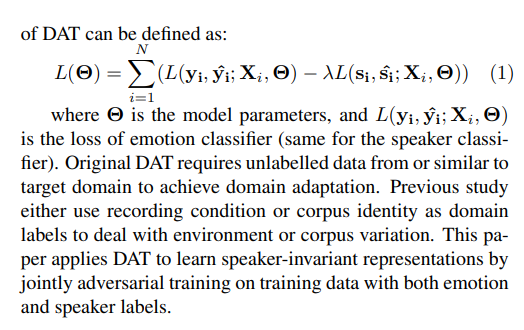I am trying to use adversarial learning from paper

Since I have implemented it, but I am confused how do I combine the two losses (my both losses here are cross entropy because I want to predict the label of emotion sample and trying to make the latent embeddings speaker invariant using the SpeakerInvariant Adversarial Training ) here one from Emotion Classifier and other from SpeakerInvariant.

The second question is how do apply gradient reversal in the SpeakerInvariant Layer in the backward step.

The loss function is as:``````class Adversarial(nn.Module):
def __init__(self):
self.cnn = CNN()
self.emotion_classfier = EmotionClassfier()
self.speaker_invariant = SpeakerInvariant()

def forward(self, input):
input         = self.cnn(input)
input_emotion = self.emotion_classfier(input)
input_speaker = self.speaker_invariant(input)
"""First confusion is here, how shall handle it here and then how to implemenet this loss function  """
return input_stutter, input_speaker

class EmotionClassfier(nn.Module):
def __init__(self):
super(EmotionClassfier, self).__init__()
self.fc1 = nn.Linear(128, 64)
self.fc2 = nn.Linear(64, 5)     #5 here is number of emotion classes
self.relu = nn.ReLU()

def forward(self, input):
input = input.view(input.size(0), -1)
input = self.fc1(input)
input = self.relu(input)
input = self.fc2(input)
input = F.softmax(input,1)
return input

class SpeakerInvariant(nn.Module):
def __init__(self):
super(SpeakerInvariant, self).__init__()
self.fc1 = nn.Linear(128, 64)
self.fc2 = nn.Linear(64, 11)   #11 here is number of speakers/labels
self.relu = nn.ReLU()

def forward(self, input):
input = input.view(input.size(0), -1)
input = self.fc1(input)
input = self.relu(input)
input = self.fc2(input)
input = F.softmax(input,1)
return input
``````

@ptrblck